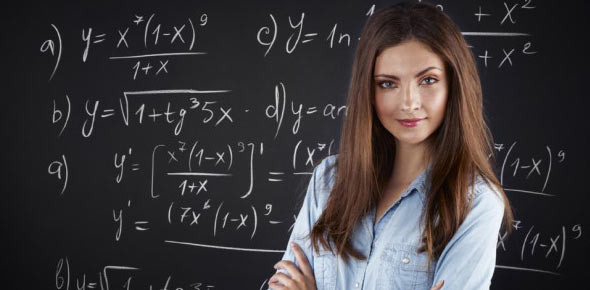# 5.4b Statistics - Independent And Dependent Events

15 Questions | Attempts: 181
ShareSettings5. 4b Statistics - Independent and Dependent Events

• 1.
1.      Write whether the events listed in the following question are either “independent” or “dependent”.  Find the probability of drawing a queen and then a second queen from a standard deck of 52-cards when you DO NOT replace the first card before selecting the second card.
• 2.
1.      Write whether the events listed in the following question are either “independent” or “dependent”.  Find the probability of drawing a heart and then a diamond from a standard deck of 52-cards when you DO NOT replace the first card before selecting the second card.
• 3.
1.      Write whether the events listed in the following question are either “independent” or “dependent”.  The owner of a one-man lawn mowing business owns four old and unreliable riding mowers.  As long as one of the four is working he can stay productive.  From past experience, one of the mowers is unusable 10 percent of the time, one 9 percent of the time, one 27 percent of the time and one 11 percent of the time.  Find the probability that all three mowers are unusable on a given day.
• 4.
1.      Write whether the events listed in the following question are either “independent” or “dependent”.  You are playing a game that involves drawing 3 numbers from a hat.  There are 20 pieces of paper numbered 1 to 20 in the hat.  Each number is replaced after it is drawn.  What is the probability that each number is greater than 15 or less than 4?
• 5.
1.      Write whether the events listed in the following question are either “independent” or “dependent”.  You are playing a game that involves drawing 3 numbers from a hat.  There are 20 pieces of paper numbered 1 to 20 in the hat.  Each number is replaced after it is drawn.  What is the probability that each number is greater than 15 or less than 4?
• 6.
1.      Find the probability of drawing a queen and then a king from a standard deck of 52-cards when you replace the first card before selecting the second card.
• 7.
1.      Find the probability of drawing a heart and then a diamond from a standard deck of 52-cards when you DO NOT replace the first card before selecting the second card.
• 8.
1.      Find the probability of drawing a heart and then a king from a standard deck of 52-cards when you replace the first card before selecting the second card.
• 9.
1.      Find the probability of drawing a heart and then a king from a standard deck of 52-cards when you DO NOT replace the first card before selecting the second card.  (Assuming the king of hearts is not the heart originally selected)
• 10.
1.      Find the probability of drawing a queen and then a second queen from a standard deck of 52-cards when you DO NOT replace the first card before selecting the second card.
• 11.
1.      Find the probability of drawing a heart and then a diamond from a standard deck of 52-cards when you DO NOT replace the first card before selecting the second card.
• 12.
1.      The owner of a one-man lawn mowing business owns four old and unreliable riding mowers.  As long as one of the four is working he can stay productive.  From past experience, one of the mowers is unusable 10 percent of the time, one 9 percent of the time, one 27 percent of the time and one 11 percent of the time.  Find the probability that all three mowers are unusable on a given day.
• 13.
1.      You are playing a game that involves drawing 3 numbers from a hat.  There are 20 pieces of paper numbered 1 to 20 in the hat.  Each number is replaced after it is drawn.  What is the probability that each number is greater than 15 or less than 4?
• 14.
1.      You are playing a game that involves drawing 3 numbers from a hat.  There are 20 pieces of paper numbered 1 to 20 in the hat.  Each number is replaced after it is drawn.  What is the probability that each number is greater than 15 or less than 4?
• 15.
1.      You and three friends go to a restaurant and order a sandwich.  The menu has 20 types of sandwiches and each of you is equally likely to order any type.  What is the probability that each of you orders a different type?

## Related TopicsBack to top
×

Wait!
Here's an interesting quiz for you.